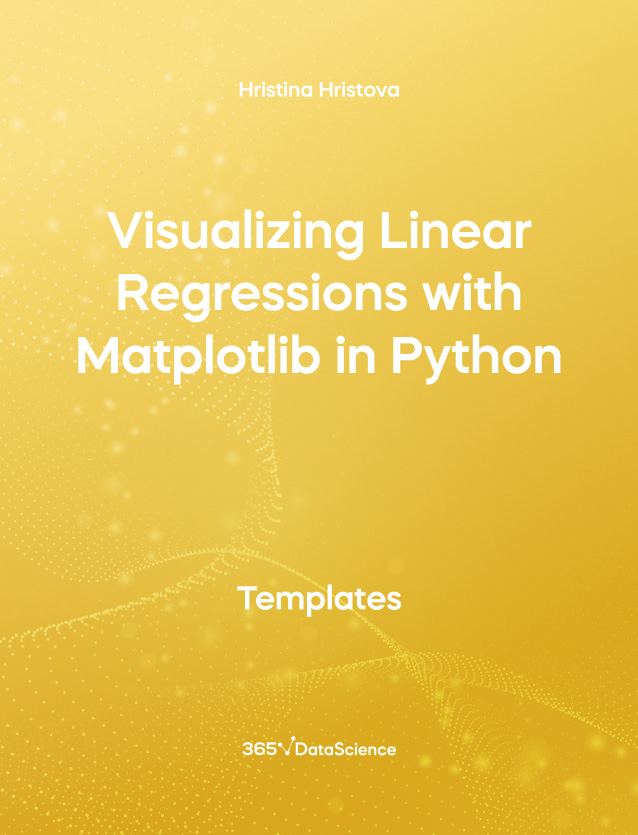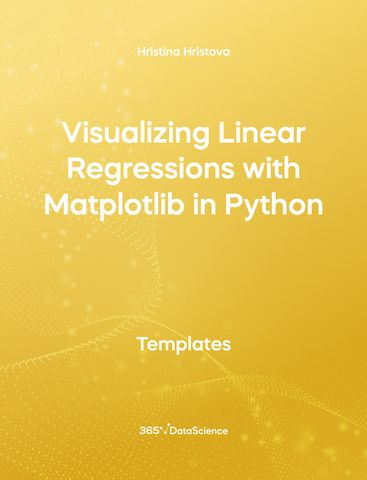# Visualizing Linear Regressions with Matplotlib in Python TemplateHristina Hristova

Course Author
The Visualizing Linear Regressions with Matplotlib in Python template demonstrates how to plot the regression line of a linear regression model onto the data. We go through the steps of loading the data from a .csv file, then mapping dummy variables onto numerical values, performing a linear regression using statsmodels and, finally, visualize what we have created. Some other related topics you might be interested in are Regression Summary Table with statsmodels in Python, Predictions with statsmodels in Python, Linear Regression Model in Python - predictions versus targets. eeee You can now download the Python template for free. The Visualizing Linear Regressions with Matplotlib in Python template is among the topics covered in detail in the 365 Data Science program.

## Who is it for

Data Analysts, Machine Learning Engineers, Data Scientists and any person who wants to visualize linear regressions with Matplotlib in Python will find this open-access Python template really helpful.